# Tools for constructing SV Matrix

Here you will find tools for calculating the Linda index (L), modified indices of concentration (CRSV) and Hall-Tideman (HTSV), as well as graphical construction of the SV matrix.

To construct the SV matrix, it is necessary to assess the presence and market share of the dominant group in all studied markets or industries with the help of the Lind Index (L), to calculate the corresponding CRSV and HTSV indices, and to plot the corresponding points on the matrix.

Here you can find:

1. What data is needed to construct the SV Matrix?

2. How to calculate the Lind index?

3. How to calculate the CRSV index?

4. How to calculate the HTSV index?

5. Excel template for calculating the indices and constructing SV Matrix

6. Template for constructing the SV Matrix

7. An example of constructing the SV Matrix

1. What data is needed to construct the SV matrix?

To construct the SV matrix, you need data on the market size and market shares of the largest companies or other data to calculate the market shares (e.g., the company's revenue and market volume in absolute terms). Usually, the data on the revenue of the largest 5 - 10 companies and the estimation of the market size is available in open sources of information. For some markets (e.g., oil, metal), you can use the exchange prices and shipment/production volumes to estimate the market share.

2. How to calculate the Lind index?

The SV Matrix can be applied only for markets or industries with identified group dominance. To determine the size of the dominant group, we can use the Lind Index (L), calculated using the following formula, proposed by Remo Linda in 1976 (Linda 1976, 19):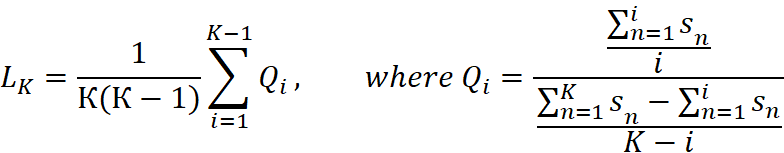Where

K is the number of the large sellers;

i is the number of leading sellers among K large sellers;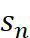- market share of the n-th seller;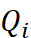- the ratio between the average market share of I sellers and the share of (K - i) sellers;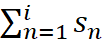- market share of i sellers (CRi);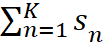- market share of K large sellers (CRk).

Accordingly, L can be expressed in terms of C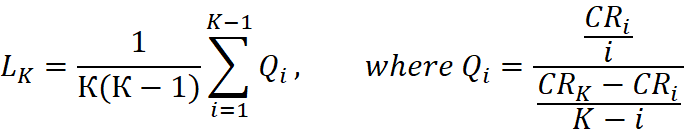or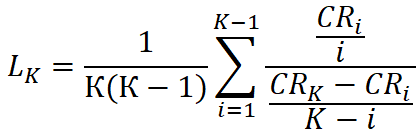To identify the group of dominant companies, the Lind Index is calculated for the 2 largest companies, then 3, then 4, and so on until the first discontinuity (Ln is < Ln+1) is found, where n companies are considered to form a dominant group. As mentioned above, usually the market share is calculated by revenue data, but if this information is not available or to increase the reliability of calculations, you can also use other comparable parameters (e.g., the number of employees, net profit, cash flow, or gross income)

You can find the Excel calculation template and examples below.

3. How to calculate the CRSV index?

СRSV is the total market share occupied by the group of dominant companies in a particular market, that is, the standard concentration ratio (CRn) for a group of n dominant companies in a particular market, where n is determined with the Lind Index (or expert method).

Thus, if the market has n dominant companies with a certain market share Si for each i-th company, then the formula for calculation the CRSV Index will be: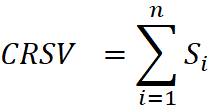4. How to calculate the HTSV index?

HTSV is a Hall-Tideman Index (HT) calculated for the identified group of n dominant companies (in accordance with the theory of economic dominance (for more information see «Publications») we will call them alpha companies) and modified to compare groups of alpha companies of different sizes (since it is impossible to use the classical Hall-Tideman index to compare the markets with each other due to the different minimum HT values for a different number of dominant companies).

Let's consider it in more detail.

The classic Hall-Tideman Index is calculated based on the ranks of firms in the market, where the largest (usually by revenue) firm has rank 1, the next 2, etc., and their market shares: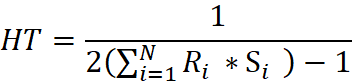where Ri is the rank of a company in the market (1,2,3, etc.), Si is the market share of the respective company, and N is the number of companies in the market.

If there is a dominant group of n alpha companies in the market, then for calculating HTSV it is necessary (1) to normalize the market shares of each alpha company by the size of the entire dominant group of n alpha companies and to calculate HTn for them according to the standard formula for n companies, and then (2) to multiply the resulting number by the correction factor.

Let's take a closer look at these steps:

4.1 To normalize HT

To normalize HT, we need to calculate the market share for each of the n alpha companies only from the consolidated market share of all alpha companies. To do this, we can divide the Si market share of each alpha company by the CRSV index (the sum of the alpha companies' market shares). Then the normalized Hall-Tydeman index - let's call it HTn - looks like this: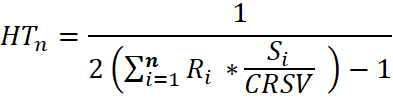Note that the summation is only for n alpha companies, not all N companies in the market, as in the previous classic HT formula.

4.2 To modify HTn to HTSV

If HT is calculated according to the standard formula, then it will give values in the range from 1/n to 1.  If the dominant group has two companies, then HT will vary from 1/2 to 1, and if there are seven companies, then from 1/7 to 1. To be able to compare markets with different numbers of dominant companies, we introduce a correction factor so that the final HTSV index changes for any n in the range from 0 up to 1: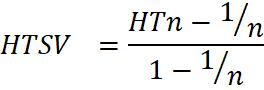Accordingly, HTSVn can be expressed in terms of classic HT, calculated based on the normalized market shares of n alpha companies: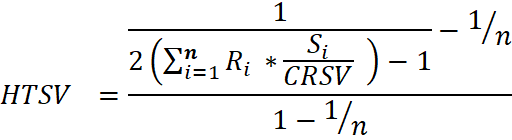The formula may seem complicated, but it is easy to calculate through Excel or similar programs.

5. Excel template for calculating the indices and constructing the SV matrix

Here you can download a template with instructions for constructing an SV matrix in Excel. For experienced users who want to check the calculations (we will be grateful!), the password for hidden sheets is 123. If you have any questions about the matrix, write us.

6. Additional template for visualizing the SV matrix

Soon you will find here additional templates for visualizing the SV matrix. For now, we recommend using the charts from the Excel template above.

7. Сode for R for building the SV matrix in the RStudio

Here you can download code for R for building the SV matrix in the RStudio. To test the code, you can use this data set, we tested code on. If you have questions about using the code, contact this e-mail.